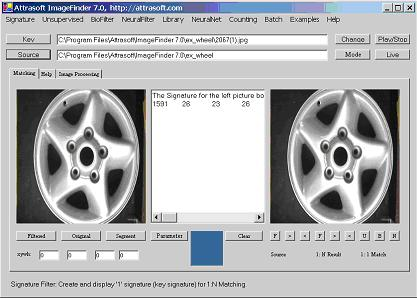[Home][12 Image Signatures][12.4 Key Signature]

## 12.4   Key Signature

You can collect the signature(s) of the left image, the right image, and all of the images in a folder. To compute the signature of an image:

•    Click the Key button to select a key image or sample image;
•    Click the Signature/Left Signature (Key) to compute the signature.

Example. Left Signature.

To continue from Figure 3.4, click the Clear button to clear the text box. Click Signature/Left Signature (Key) and you will see Figure 12.2.Figure 12.2  Click Signature/Left Signature (Key).

The Signature looks like:

1591    26  23  26  23

Similarly, click Signature/Right Signature and you will get an image signature for the right image.

In 1:1 Matching or 1:N Matching, the signatures are computed behind the screen; you do not need to use the signature directly. The menu item Signature/Left Signature (Key) shows you the mechanism behind the screen so you can see what a signature looks like.

Now we implement these two menu items. Double click these menu items and enter:

private void menuItem2_Click(object sender, System.EventArgs e)

{

}

Here, mainMenuToAPI is an object, which will implement all functions. As we discussed in the last chapter, the main form simply links menu items to functions in the mainMenuToAPI object. The implementation is:

public bool signature_Key_Signature ( string key)

{

if ( ! System.IO .File .Exists (key) )

{

appendText ( "Please enter a valid Key!\n");

return false;

}

int x =0, y = 0, w =0, h = 0;

try

{

x = int.Parse (f.textBox3.Text );

y = int.Parse (f.textBox4.Text );

w = int.Parse (f.textBox5.Text );

h = int.Parse (f.textBox6.Text );

}

catch

{

setText ("Invalid integers!\n");

x= 0;

y = 0;

w = 0;

h = 0;

return false;

}

script.ImageSignature

= script.signatureFilter.getSignature (key, "1 of 1:N", x, y, w, h );

if ( script.imageSignature != null )

{

setLine ("The Signature for the left picture box:");

appendLine (script.imageSignature.toString () );

return true;

}

else

return false;

}

The following section of code simply makes sure the key image exists:

if ( ! System.IO .File .Exists (key) )

{

appendText ( "Please enter a valid Key!\n");

return false;

}

The following section of code computes (x, y, w, h) of the key segment:

int x =0, y = 0, w =0, h = 0;

try

{

x = int.Parse (f.textBox3.Text );

y = int.Parse (f.textBox4.Text );

w = int.Parse (f.textBox5.Text );

h = int.Parse (f.textBox6.Text );

}

catch

{

setText ("Invalid integers!\n");

x= 0;

y = 0;

w = 0;

h = 0;

return false;

}

The following section of code computes the signature of the key segment:

script.ImageSignature

= script.signatureFilter.getSignature (key, "1 of 1:N", x, y, w, h );

In this statement, the script object contains all of the objects required for the ImageFinder project. In particular, script.ImageSignature is the image signature object; and script.signatureFilter is the signature filter object. The parameter, "1 of 1:N", is the ID, which we arbitrarily assigned to the key image. The following section of code prints the signature of the key segment in the text window:

if ( script.imageSignature != null )

{

setLine ("The Signature for the left picture box:");

appendLine (script.imageSignature.toString () );

return true;

}

The menu item, Signature/Right Signature, is implemented in a similar fashion.